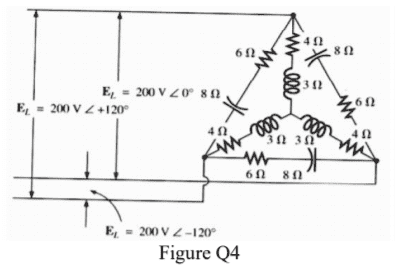• Jinium

## Homework StatementBased on Fig. 4,
ZΔ=6+8j Ω
ZY=4+3j Ω
EL=200V

Show that the equivalent delta-connected load is 7.846 - 2.77j

ZY=(1/3)ZΔ

## The Attempt at a Solution

Here's my thought process:
For part (i), attempt to convert Y to Δ,
So using the relevant equation above
ZΔ of transformed ZY is 12 + 9i
However, that's not done because I need to combine the two delta loads.
Hence, I use the product/sum rule to obtain the combined delta:
((12+9i)(6+8j))/((12+9i)+(6+8j))
which obtained 4.1598 + 4.405j which is wrong

I also used https://www.physicsforums.com/threads/delta-and-star-transformation-ac-circuits.791979/ as guidance for this problem but can't quite point out where my mistake is.

#### Attachments

The existing impedance of the delta portion is 6 - j8. You used 6 + j8.

Try that.

•Jinium
magoo said:
The existing impedance of the delta portion is 6 - j8. You used 6 + j8.

Try that.

Worked ! Guess I haven't studied enough. I should go read up on the positive and negative conventions of these circuits. I was not aware it could be a negative just from the diagram.

Thanks, magoo !

EDIT: Got it. It's negative on 8j because it's a capacitor. It would be positive if it were and inductor instead.

Last edited:
•jim hardy